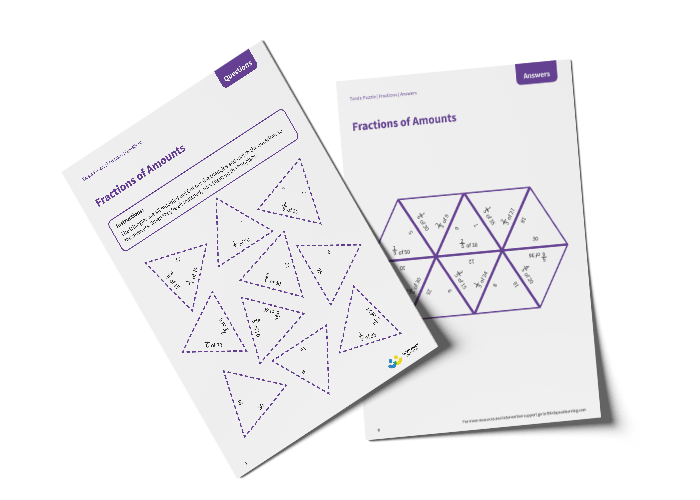# Tarsia Puzzle Fractions of Amounts (Year 3)

The triangles in the tarsia puzzle have all been mixed up. In this fractions of amounts tarsia puzzle, pupils will need to match the fraction of amount question to the correct answer to create one large hexagon.

This Year 3 fractions of amounts tarsia puzzle worksheet contains:

• One question sheet
• One template sheet

Year 3

Fractions